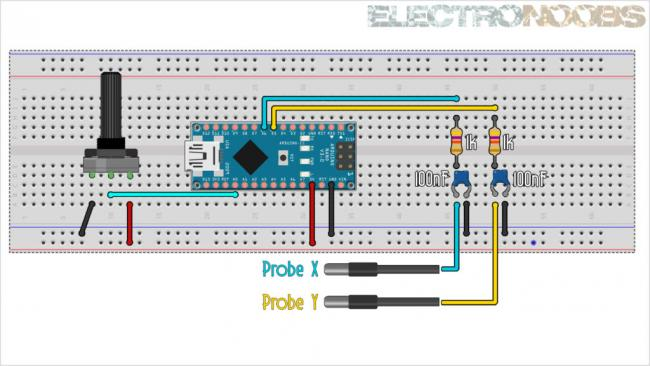﻿

# Рисуем на осциллографе с помощью Arduino

При помощи контроллера Arduino можно выводить простые рисунки на экране осциллографа.
Для этого, нужно задействовать всего два вывода от Arduino Nano для генерации быстрого ШИМ-сигнала. Затем, сигнал нужно отфильтровать при помощи простого фильтра из резистора (1k) и конденсатора (100nF) (RC-фильтр).
И всё — можно рисовать фигуры на осциллографе в режиме XY.
Единственное ограничение — формы должны быть простыми и заканчиваться в том же месте, где они начинаются (без самопересечений).

СхемаСкетч для Arduino
``````
//Inputs/Outputs
int X_pin = 6;
int Y_pin = 5;
int Pot = A0;

int point_delay = 1000;         //Delay between loops in us
#define how_many_vertices  19   //Put here the amount of vertices that you ahve
//Coordinates for the X axis (remember, up to 254 coordinates)
byte x_axis[how_many_vertices] = {
9,9,3,9,4,9,6,9,8,10,12,11,14,11,16,11,17,11,11};
//Coordinates for the Y axis
byte y_axis[how_many_vertices] = {
3,6,6,10,10,14,14,17,17,19,17,17,14,14,10,10,6,6,3};

void setup() {
pinMode(X_pin,OUTPUT);      //Define the pin as output
pinMode(Y_pin,OUTPUT);      //Define the pin as output
pinMode(Pot,INPUT);         //Define the pin as input for the potentiometer

//Now we adjust PWM frequency using Prescale_factor
TCCR0A =  (TCCR0A & B10100011 + TCCR0A | B10100011);  //(1<<COM0A1 | 0<<COM0A0 | 1<<COM0B1 | 0<<COM0B0 | 1<<WGM01 | 1<<WGM00);
TCCR0B =  (TCCR0B & B00000001 + TCCR0B | B00000001);  //(0<<FOC0A | 0<<FOC0B | 0<<WGM02 | 0<<CS02 | 0<<CS01 | 1<<CS00);
TIMSK0 =  (TIMSK0 & B11111000);                       //(0<<OCIE0B | 0<<OCIE0A  | 0<<TOIE0);

}

void loop() {
unsigned char loopcount;
{
point_delay = map(analogRead(Pot),0,1024,10,2000);                //Read analog value adn map it to a range between 10 and 2000us
for(loopcount = 0; loopcount < how_many_vertices; loopcount++)
{
analogWrite(X_pin,x_axis[loopcount]);                           //In this "for" we change the analog PWM width according to X axis and Y axis
analogWrite(Y_pin,y_axis[loopcount]);
delayMicroseconds(point_delay);                                 //Add the delay
}
}
}
``````

Ссылки
Arduino XY plot draw oscilloscope christmas tree

По теме
ШИМ — Широтно-Импульсная Модуляция
Практическое программирование Arduino — Аналоговый ввод – осциллограф

Arduino
Ардуино что это и зачем?
Arduino, термины, начало работы
КМБ для начинающих ардуинщиков
Состав стартера (точка входа для начинающих ардуинщиков)
• 0
• 28 декабря 2018, 09:15

### Комментарии (0)

RSS свернуть / развернуть

Только зарегистрированные и авторизованные пользователи могут оставлять комментарии.